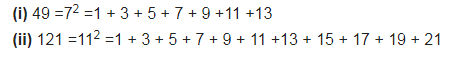# (i) Express 49 as the sum of 7 odd numbers

(i) Express 49 as the sum of 7 odd numbers.
(ii) Express 121 as the sum of 11 odd numbers.